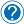# The ternary conditional operator

## The ternary conditional operator

In computer programming, `?:` is a ternary operator that is part of the syntax for a basic conditional expression in several programming languages. It is commonly referred to as the conditional operator, inline if (iif), or ternary if.

## Important because…

Every good developer knows that less code that do something is better than more code that do the same thing, right ? When writing large code you need to structure it to be as readable and as small as you can in order to take little time in execution and 100%  understood by the other developers that are working on it.

It also allows you to check for the existence of a variable and assign a value accordingly. This is very useful when you are dealing with \$_GET, \$_POST or \$_SESSION variables, because you don’t know if the incoming variable exist.

## Usage

The condition is evaluated true or false as a boolean expression. On the basis of the evaluation of the boolean condition, the entire expression returns “value if true” if condition is true, but “value if false” otherwise. Usually the two sub-expressions value_if_true and value_if_false must have the same type, which determines the type of the whole expression. Here is the format of the ternary conditional operator:

This is interpreted as this:

## Example in php

The above code is written on one line and do so much stuff. First  it uses the isset() function to check if the “id” from \$_GET exists. If it does, it simply returns its value. However, if it does not exist the operator returns false. The value that the operator returns is then assigned to the variable \$id. So, basically, if \$_GET[‘id’] exists then \$id = \$_GET[‘id’], however if it does not exist then \$id = false.

The operator can be useful in a number of applications, and helps you to avoid loads of unnecessary if statements.Request an article ←